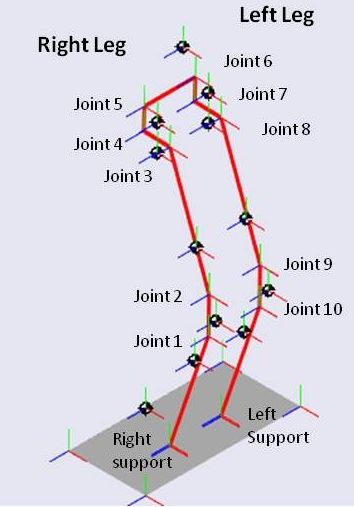## Jacobian model in SimMechanics (MatLab/Simulink)

We did a first approach to build the jacobian dynamic model in MatLab 2009b / Simulink using the blocks of SimMechanics Toolbox.

In this first approach, we used he properties of the structure and body parts with a robot pose approximated at the equilibrium point.

The SimMechanics blocks needs some parameters (center of mass and inertia matrix of each body part and joint coordinates) that are obtained in report “Mass Properties of Jacobian – equilibrium point

To view the simulation, download the model, start the Matlab 2009b (the model could not open in prior versions), open the Simulink model and click in “Start” and view the structure in the machine for model.This model show the structure of joints and allow you to move each one. However, at a moment the structure are still constrainted in the contact point with the floor.

This is a problem (the contact with floor) that we must to solve because the SimMechanics doesn’t have any block to simulate the floor. Thus, we created contact points (one for each leg) and the force applied by the floor (reaction force).

This is a limitation of SimMechanics that we are trying to minimize in order to simulate the movement of the bipede robot over the floor.